# How to Compare Two Columns to Find Missing Value (Unique Value) in Excel

Suppose we have two lists, list A contains all students, list B only contains students who passed exam. So, list A is longer than list B, and students in list B are all included in list A. If we want to find out which student failed the exam, we can though compare the two list and find out the missing value to confirm the name list. Actually, in our work we often meet the situations that we need to compare two lists and find out the missing values. In this tutorial, we will help you to find out missing values via two ways, the first one is by Conditional Formatting function in excel, the second one is by using formula with VLOOKUP function.

Precondition:

Prepare two lists. List A contains all students. List B contains partial of them.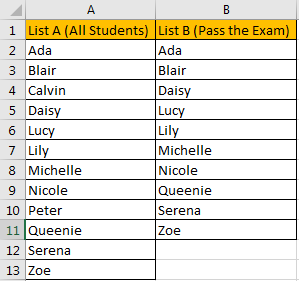## Method 1: Compare Two Columns to Find Missing Value by Conditional Formatting

Step 1: Select List A and List B.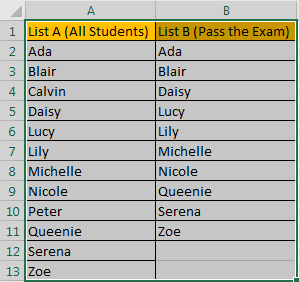Step 2: Click Home in ribbon, click Conditional Formatting in Styles group.Step 3: In Conditional Formatting dropdown list, select Highlight Cells Rules->Duplicate Values.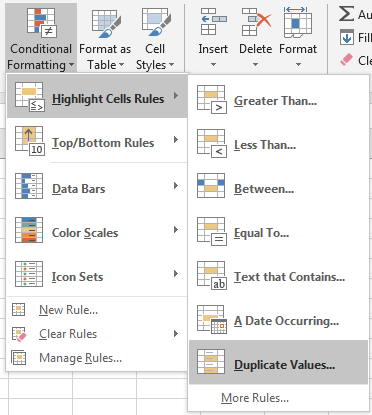Step 4: In Duplicate Values dialog, select Unique in dropdown list. Keep default value in values with dropdown list. Then click OK.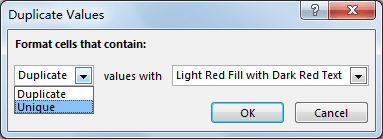Step 5: Verify that unique values are marked with dark red properly.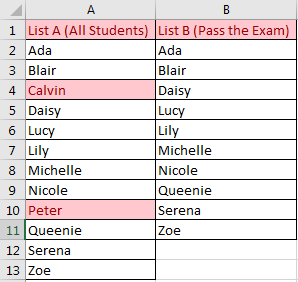This way can be used in finding unique values from two lists.

## Method 2: Compare Two Columns to Find Missing Value by Formula

Insert a new column between List A and List B.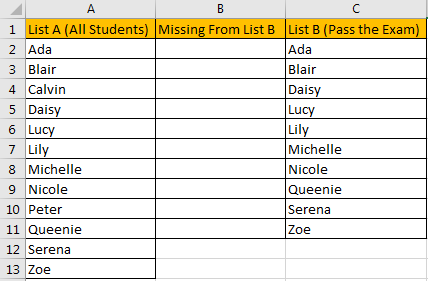Step 1: In B2 enter the formula =ISERROR(VLOOKUP(A2,\$C\$2:\$C\$11,1,FALSE)). VLOOLIP function can help us to look up match value from List B. ISERROR function is used for checking whether match value exists or not, it returns TRUE or FALSE.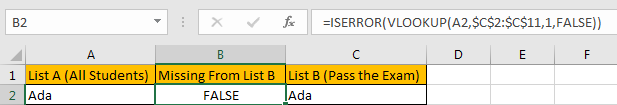Step 2: Drag the fill handle down. Verify that B4 and B10 are filled with TRUE.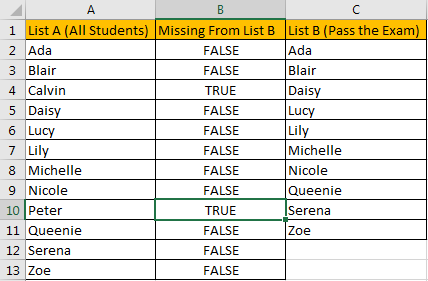So, in List A, Calvin and Peter are students who failed in exam.

Step 3: If you want to show student name in new list directly, you can update formula =IF(ISERROR(VLOOKUP(A2,\$C\$2:\$C\$11,1,FALSE)),A2, “”).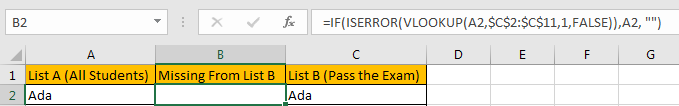Step 4: After applying above formula for the following cells, missing value will display in new list directly.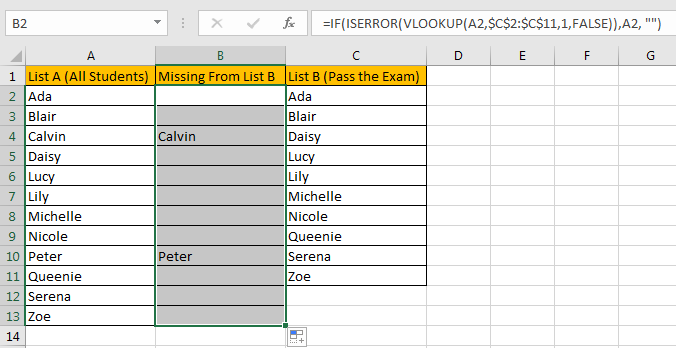### Related Functions

• Excel IF function
The Excel IF function perform a logical test to return one value if the condition is TRUE and return another value if the condition is FALSE. The IF function is a build-in function in Microsoft Excel and it is categorized as a Logical Function.The syntax of the IF function is as below:= IF (condition, [true_value], [false_value])….
• Excel VLOOKUP function
The Excel VLOOKUP function lookup a value in the first column of the table and return the value in the same row based on index_num position.The syntax of the VLOOKUP function is as below:= VLOOKUP (lookup_value, table_array, column_index_num,[range_lookup])….
• Excel ISERROR function
The Excel ISERROR function used to check for any error type that excel generates and it returns TRUE for any error type, and the ISERR function also can be checked for error values except #N/A error, it returns TRUE while the error is #N/A. The syntax of the ISERROR function is as below:= ISERROR (value)….

Related Posts

Build Hyperlink With VLOOKUP in Excel

You might have come across a task in which you were assigned to build hyperlinks, which seems very easy, and if you are new to excel or don't have enough experience with it, then you might wonder about doing this ...

Calculate Total Cost with Excel VLOOKUP Function

In today's article we will show you how to calculate the total cost for a given weight using the Excel VLOOKUP function. This function will help us to find the appropriate unit price for that weight and then we can ...

Basic Price Discount Calculation with Excel VLOOKUP Function

We often encounter product discounts in our shopping. Depending on the level of spending, the mall will offer different percentages of discounts. Usually, the more you spend, the bigger the discount, while the less you spend, the smaller the discount. ...

Calculate Grades With VLookup in Excel

Why Should You Calculate Grades With VLookup Excel? If you're looking for a simple way to find out a student's grades, VLOOKUP Excel can do the trick. This function uses a lookup table to find the values and sort them ...

Convert State Names To Abbreviations

Assume that you got a task to convert the full state's name into the abbreviations in MS Excel, and for doing this task, you might choose to do it manually, which is an acceptable way only if you don't have ...

Sidebar